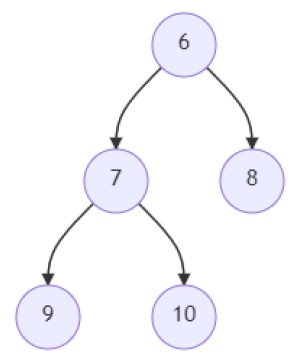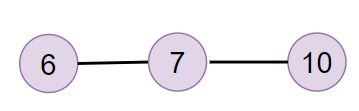# Program to find out if a linked list is present in a given binary tree in Python

Suppose we are given a binary tree that has a root node 'root' and a linked list that has a head node 'head'. We have to find out if that linked list exists in that binary tree. If a set of nodes in the tree have links with each other in order as a linked list, and if that order is similar to that of the provided linked list, then we return 'True' or otherwise, we return 'False'.

So, if the input is likeTreethen the output will be True.

To solve this, we will follow these steps −

• arr := a new list
• size := size of arr
• temp_arr := an array of size (size + 1) initialized with -1
• Define a function helper() . This will take root, val
• if val >= size, then
• return True
• if root is similar to None, then
• return False
• val := val + 1
• while val > 0 and value of root is not same as arr[val - 1], do
• val := temp_arr[val - 1] + 1
• if helper(left of root, val) or helper(right of root, val) is True, then
• return True
• return False
• while start is not same as null, do
• add the value of start at the end of arr
• start := next of start
• for node in range 1 to size + 1, do
• temp_arr[node] := temp_arr[node - 1] + 1
• while temp_arr[node] > 0 and arr[node - 1] is not same as arr[temp_arr[node] - 1], do
• temp_arr[node] := temp_arr[temp_arr[node] - 1] + 1
• return helper(root, 0)

## Example

Let us see the following implementation to get better understanding −

class TreeNode:
def __init__(self, val, left=None, right=None):
self.val = val
self.left = left
self.right = right

class ListNode:
def __init__(self, val, next=None):
self.val = val
self.next = next

def insert(temp,data):
que = []
que.append(temp)
while (len(que)):
temp = que
que.pop(0)
if (not temp.left):
if data is not None:
temp.left = TreeNode(data)
else:
temp.left = TreeNode(0)
break
else:
que.append(temp.left)

if (not temp.right):
if data is not None:
temp.right = TreeNode(data)
else:
temp.right = TreeNode(0)
break
else:
que.append(temp.right)

def make_tree(elements):
node = TreeNode(elements)
for element in elements[1:]:
insert(node, element)
return node

def make_list(elements):
for element in elements[1:]:
while ptr.next:
ptr = ptr.next
ptr.next = ListNode(element)

arr = []
while start:
arr += (start.val,)
start = start.next
size = len(arr)
temp_arr = [-1] * (size + 1)
for node in range(1, size + 1):
temp_arr[node] = temp_arr[node - 1] + 1
while temp_arr[node] > 0 and arr[node - 1] != arr[temp_arr[node] - 1]:
temp_arr[node] = temp_arr[temp_arr[node] - 1] + 1
def helper(root, val):
if val >= size:
return True
if not root:
return False
val += 1
while val > 0 and root.val != arr[val - 1]:
val = temp_arr[val - 1] + 1
if helper(root.left, val) or helper(root.right, val):
return True
return False
return helper(root, 0)

root = make_tree([6, 7, 8, 9, 10])
print(solve(root, head))
root = make_tree([6, 7, 8, 9, 10])
print(solve(root, head))
True